# Merge Sort

Nov. 11, 2018 7946

This post first explains the Merge Sort algorithm and then focus on writing the algorithm in C, Python and Java.

Merge Sort works on divide and conquer, it first breaks an array into smaller arrays and then recursively combines and sorts them simultaneously.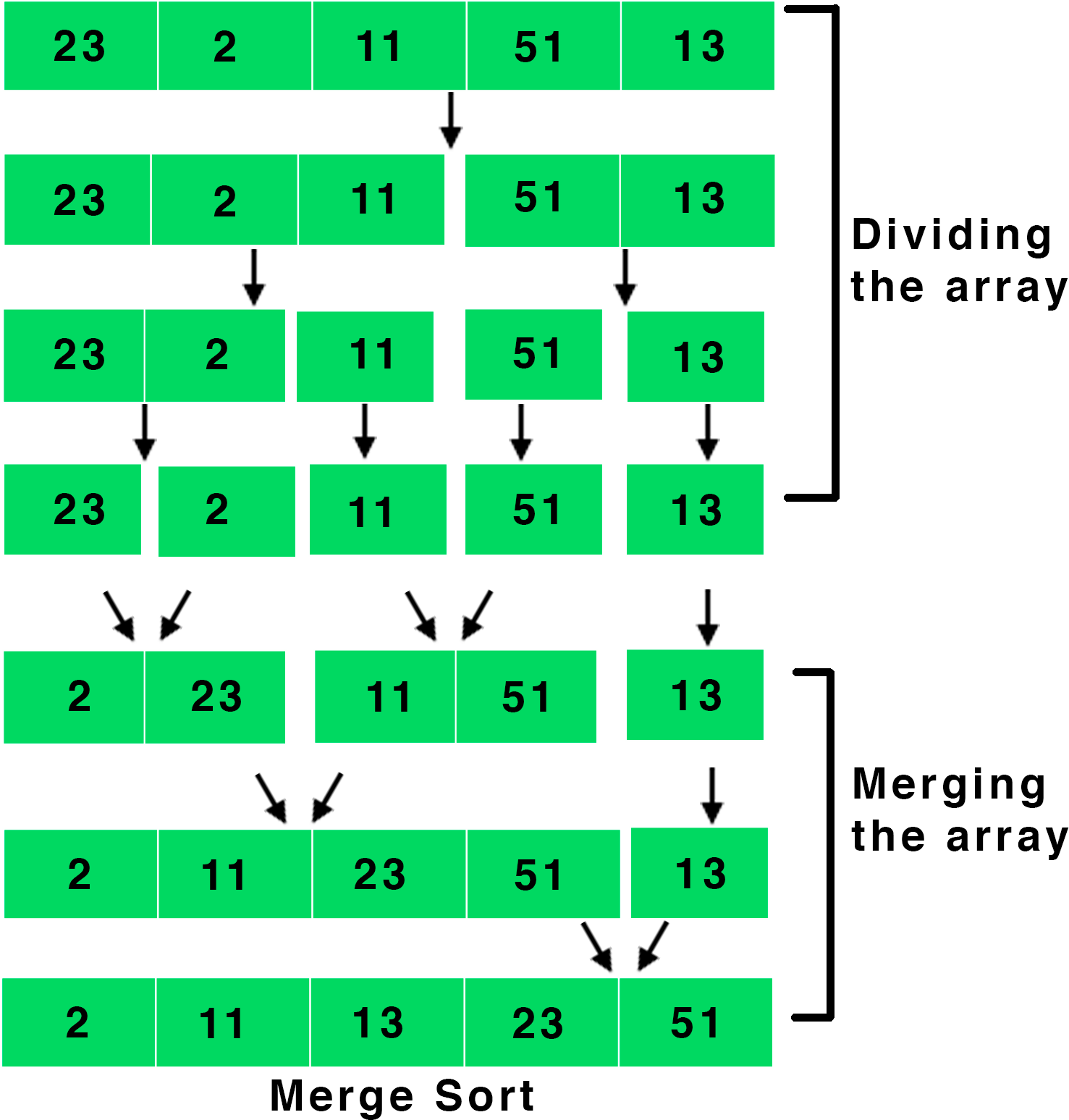To write the algorithm, we first need to make a function which will break the array into smaller arrays and then one more function to combine these arrays back into the bigger array while sorting them. So, let's start by making the first function to break the array into smaller arrays.

To break an array into smaller arrays, we will make a function which will take the array and the start and the last indices as it parameters i.e., merge_sort(array, left, right). Now, we will recursively break the array from the middle as shown in the picture given below.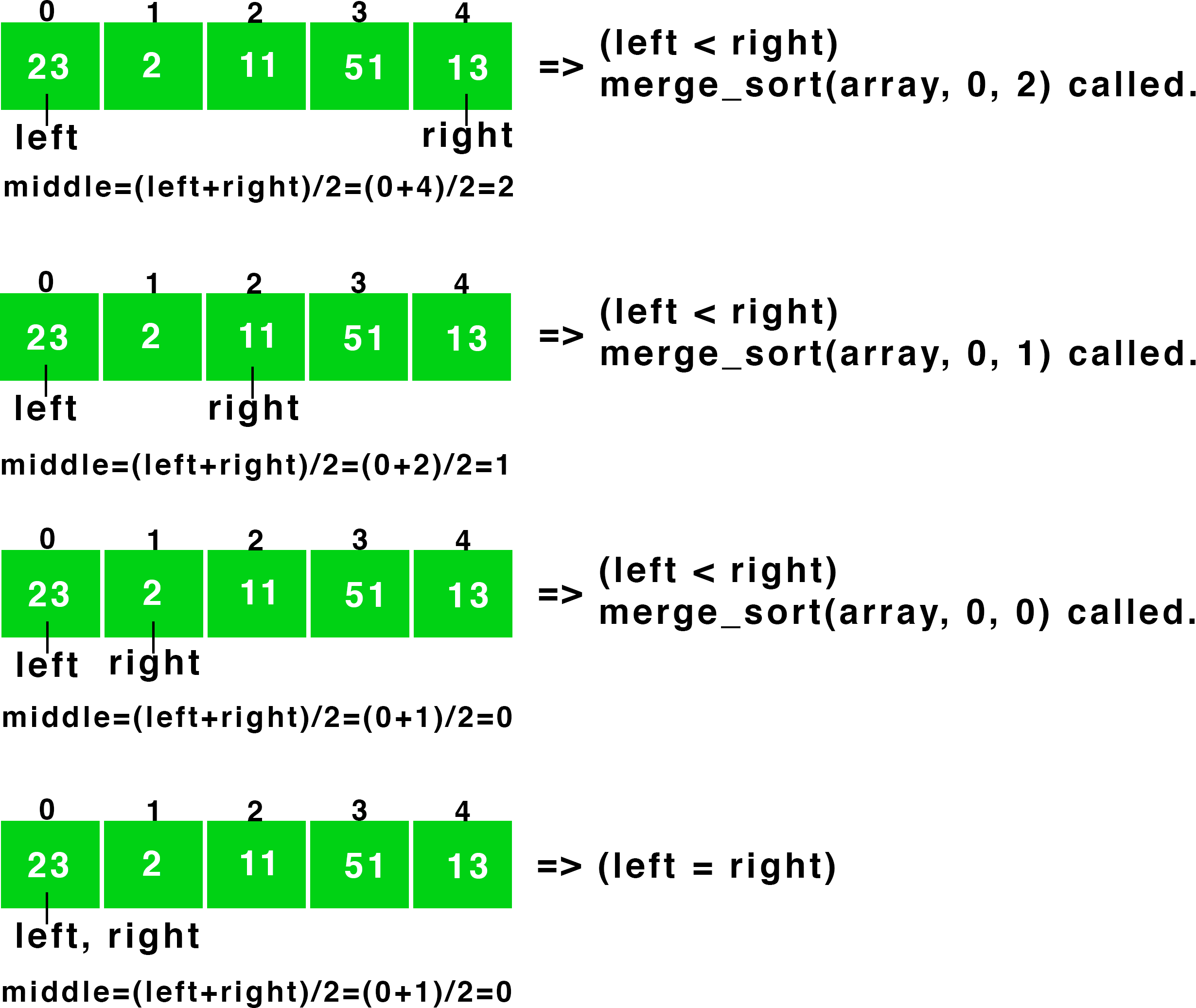From the above picture, it is clear that we are first getting the middle element by (left+right)/2 and then calling the function with arguments - array, left for the starting index and middle for the last index i.e., calling merge_sort(array, left, middle) inside merge_sort(array, left, right).

Similarly, we will call the merge_sort function with arguments - array, middle+1 for the starting index and right for the last index i.e., merge_sort(array, middle+1, right) to break the right half of the array as well.

We will repeat this process until both left and right point to the same element or left < right.

The numbers in the picture given below represent the order in which the functions were executed.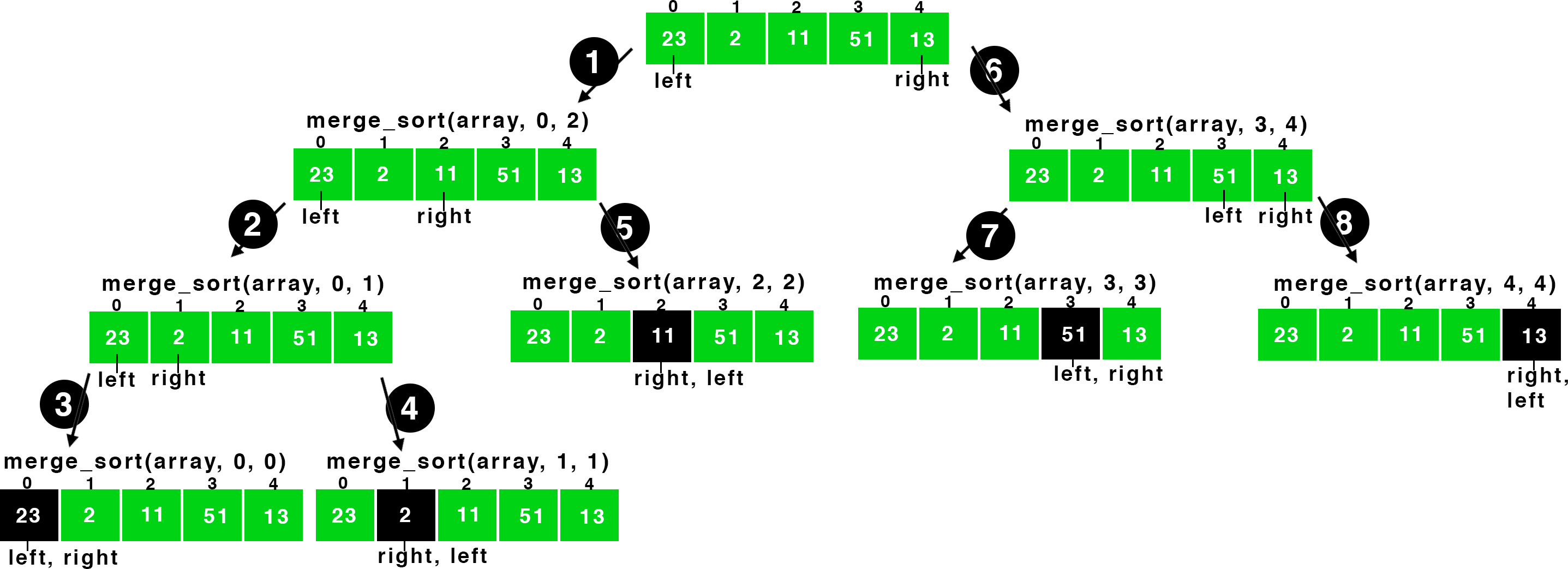Now, we can write the above concepts as:

merge_sort(array, left, right) {
if (left < right) {
merge_sort(array, left, middle);
merge_sort(array, middle+1, right);
}
}


In the above picture, after the step 3, left is equal to right and thus the compiler came out from the merge_sort(array, left, middle) function and then called merge_sort(array, middle+1, right) at the step 4.

But we are missing one thing, the function to recombine the arrays to sort them. So, let's hold the merge_sort function for a while and let's write the merge function to merge two arrays.

Before writing the function, let's look at the picture given below to understand how merging works.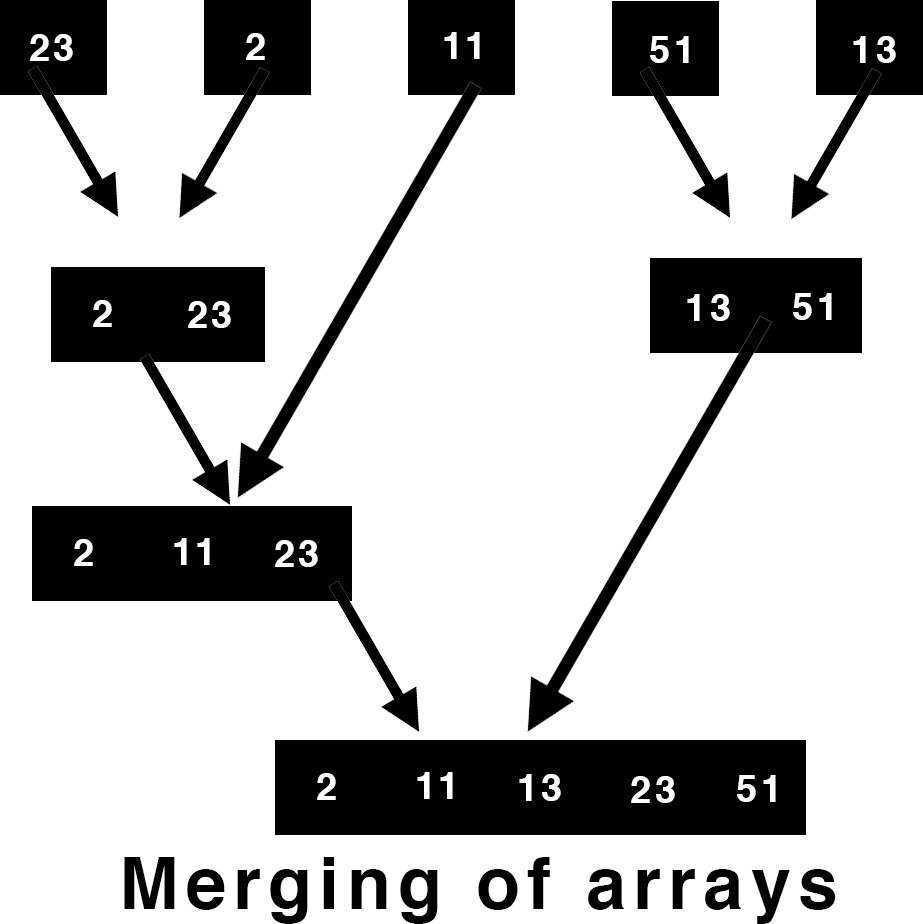From the above picture, it is clear that the function to merge will combine two arrays which are already sorted. We will do this by simply iterating over both arrays and putting the smaller element into the bigger array as shown in the animation given below.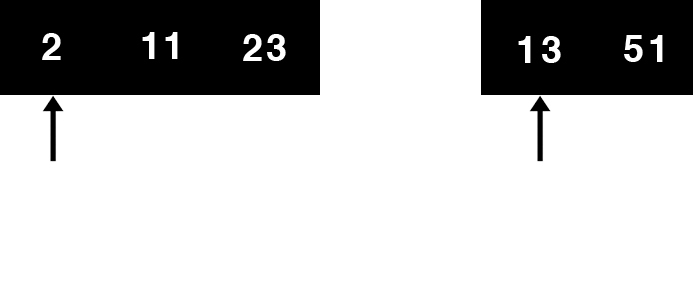Now you know the concepts behind the merging two arrays, so let's write the function to do so.

We will first store the left and the right arrays into two temporary arrays and then iterate over them and fill the smaller elements into the original array.

The parameter of the function will be - the array, left index, right index and the middle index. Our left subarray is the array from left to middle and the right subarray is from middle+1 to right and both these arrays are already sorted. Let's start writing the function:

merge(array, left, right, middle) {
size_of_temp1 = (middle-left)+1
size_of_temp2 = (right-middle)
}


size_of_temp1 and size_of_temp2 are two variables to store the size of the temporary arrays holding the left and the right subarrays respectively. Now, we need to copy the corresponding elements to these temporary arrays.

merge(array, left, right, middle) {
size_of_temp1 = (middle-left)+1
size_of_temp2 = (right-middle)

temp1 = array[left to middle]
temp2 = array[middle+1 to right]
}


Now, let's iterate over these arrays and copy the smaller elements into the original array.

merge(array, left, right, middle) {
size_of_temp1 = (middle-left)+1
size_of_temp2 = (right-middle)

temp1 = array[left to middle]
temp2 = array[middle+1 to right]

i=0
j=0
k=left

while (i < size_of_temp1 and j < size_of_temp2)
{
if (temp1[i] < temp2[j])
{
array[k] = temp1[i]
i = i+1
}

else if (temp2[j] < temp1[i])
{
array[k] = temp2[j]
j=j+1
}

k=k+1
}
}


if (temp1[i] < temp2[j]) - The element of temp1 is smaller than that of temp2. Thus, we will copy this into the original array - array[k] = temp1[i] and increase now we will move for the next element in temp1 - i=i+1. You can refer to the above animation for better understanding.

Similarly, else if will be executed if the element of temp2 is smaller than temp1.

We have to handle one more case where some elements are left either in temp1 or temp2 (As in the above animation). So, let's copy the remaining elements into the original array.

while (i<size_of_temp1)
{
ar[k] = temp1[i]
k=k+1
i=i+1
}

while (j<size_of_temp2)
{
ar[k] = temp2[j]
k=k+1
j=j+1
}


while (i<size_of_temp1) - Few elements are left in the array temp1.

while (j<size_of_temp2) - Similarly, there are elements left in the array temp2.

Now, we are done with the concepts and the codes. So, let's write the Merge Sort in C, Java and Python.

### C

#include <stdio.h>

/*
Funtion to merge the array and
do the sorting.

It is called from the merge_sort funtion.
*/
void merge(int ar[], int left, int right, int middle)
{
int size_of_temp1, size_of_temp2, i, j, k;

/*
Making two temporary arrays to copy the elements
from the index left to middle in one and middle to
right in another of the main array ar..
*/
size_of_temp1 = (middle-left)+1;
size_of_temp2 = (right-middle);

//temporary arrays
int temp1[size_of_temp1], temp2[size_of_temp1];

/*
Copying the elements from the array ar
from the index left to middle in the
first temporary array.
*/
for(i=0; i<size_of_temp1; i++)
{
temp1[i] = ar[left+i];
}

/*
Copying the elements from the array ar
from the index middle+1 to right in the
second temporary array.
*/
for(i=0; i<size_of_temp2; i++)
{
temp2[i] = ar[middle+1+i];
}

i=0;
j=0;
k=left;

/*
Now we have to merge the two arrays.
Both arrays are already sorted and have to
combine them inn such a way that the
final array is also sorted.

We will iterate over both arrays and check the
which array contains the smaller element and will
fill the main array with that element.
*/
while (i < size_of_temp1 && j < size_of_temp2)
{
//checking which array has the smaller element
if (temp1[i] < temp2[j])
{
// filling the main array with the smaller element
ar[k] = temp1[i];
// increasing the index of the temp1 array
i++;
}
//temp2 has the smaller element
else if (temp2[j] < temp1[i])
{
// filling the main array with the smaller element
ar[k] = temp2[j];
// increasing the index of the temp2 array
j++;
}
// increasing the index of the main array ar.
k++;
}

/*
copying the remaining elements
(if there are any) of temp1
to the main array ar.
*/
while (i<size_of_temp1)
{
ar[k] = temp1[i];
k++;
i++;
}

/*
copying the remaining elements
(if there are any) of temp2
to the main array ar.
*/
while (j<size_of_temp2)
{
ar[k] = temp2[j];
k++;
j++;
}
}

// merge_sort function
void merge_sort(int ar[], int left, int right)
{
/*
If left is not less than right
the base case
*/
if (left < right)
{
// middle element of the array
int middle = (left+right)/2;

/*
The merge_sort funtion
just splits the array.

Main sorting will be performed
inside the merge function.
*/
merge_sort(ar, left, middle);
merge_sort(ar, middle+1, right);

// calling the merge function
merge(ar, left, right, middle);
}
}

int main()
{
int i;

// array to be sorted
int a[] = {23, 2, 11, 51 ,13};

// calling the merge_sort function
merge_sort(a, 0, 4);

// printing the array
for (i=0; i<5; i++)
{
printf("%d ",a[i]);
}
// printing new line
printf("\n");
return 0;
}


### Python

'''
Funtion to merge the array and
do the sorting.

It is called from the merge_sort funtion.
'''
def merge(ar, left, right, middle):
'''
Making two temporary arrays to copy the elements
from the index left to middle in one and middle to
right in another of the main array ar..
'''
size_of_temp1 = (middle-left)+1
size_of_temp2 = (right-middle)

#temporary arrays
temp1 = []
temp2 = []

'''
Copying the elements from the array ar
from the index left to middle in the
first temporary array.
'''
temp1 = ar[left:middle+1]

'''
Copying the elements from the array ar
from the index middle+1 to right in the
second temporary array.
'''
temp2 = ar[middle+1:right+1]

i=0;
j=0;
k=left;

'''
Now we have to merge the two arrays.
Both arrays are already sorted and have to
combine them inn such a way that the
final array is also sorted.

We will iterate over both arrays and check the
which array contains the smaller element and will
fill the main array with that element.
'''
while (i < size_of_temp1 and j < size_of_temp2):
#checking which array has the smaller element
if (temp1[i] < temp2[j]):
# filling the main array with the smaller element
ar[k] = temp1[i]
# increasing the index of the temp1 array
i = i+1

#temp2 has the smaller element
elif (temp2[j] < temp1[i]):
# filling the main array with the smaller element
ar[k] = temp2[j]
# increasing the index of the temp2 array
j=j+1
# increasing the index of the main array ar.
k=k+1

'''
copying the remaining elements
(if there are any) of temp1
to the main array ar.
'''
while (i<size_of_temp1):
ar[k] = temp1[i]
k=k+1
i=i+1

'''
copying the remaining elements
(if there are any) of temp2
to the main array ar.
'''
while (j<size_of_temp2):
ar[k] = temp2[j]
k=k+1
j=j+1

# merge_sort function
def merge_sort(ar, left, right):
'''
If left is not less than right
the base case
'''
if (left < right):
# middle element of the array
middle = (left+right)//2

'''
The merge_sort funtion
just splits the array.

Main sorting will be performed
inside the merge function.
'''
merge_sort(ar, left, middle);
merge_sort(ar, middle+1, right)

# calling the merge function
merge(ar, left, right, middle)

if __name__ == '__main__':
# array to be sorted
a = [23, 2, 11, 51 ,13]

# calling the mergeSort function
merge_sort(a, 0, 4);

print(a)


### Java

class MergeSort {

/*
Funtion to merge the array and
do the sorting.

It is called from the merge_sort funtion.
*/
static void merge (int ar[], int left, int right, int middle) {
int size_of_temp1, size_of_temp2, i, j, k;

/*
Making two temporary arrays to copy the elements
from the index left to middle in one and middle to
right in another of the main array ar..
*/
size_of_temp1 = (middle-left)+1;
size_of_temp2 = (right-middle);

//temporary arrays
int temp1[] = new int[size_of_temp1];
int temp2[] = new int[size_of_temp1];

/*
Copying the elements from the array ar
from the index left to middle in the
first temporary array.
*/
for(i=0; i<size_of_temp1; i++)
{
temp1[i] = ar[left+i];
}

/*
Copying the elements from the array ar
from the index middle+1 to right in the
second temporary array.
*/
for(i=0; i<size_of_temp2; i++)
{
temp2[i] = ar[middle+1+i];
}

i=0;
j=0;
k=left;

/*
Now we have to merge the two arrays.
Both arrays are already sorted and have to
combine them inn such a way that the
final array is also sorted.

We will iterate over both arrays and check the
which array contains the smaller element and will
fill the main array with that element.
*/
while (i < size_of_temp1 && j < size_of_temp2)
{
//checking which array has the smaller element
if (temp1[i] < temp2[j])
{
// filling the main array with the smaller element
ar[k] = temp1[i];
// increasing the index of the temp1 array
i++;
}
//temp2 has the smaller element
else if (temp2[j] < temp1[i])
{
// filling the main array with the smaller element
ar[k] = temp2[j];
// increasing the index of the temp2 array
j++;
}
// increasing the index of the main array ar.
k++;
}

/*
copying the remaining elements
(if there are any) of temp1
to the main array ar.
*/
while (i<size_of_temp1)
{
ar[k] = temp1[i];
k++;
i++;
}

/*
copying the remaining elements
(if there are any) of temp2
to the main array ar.
*/
while (j<size_of_temp2)
{
ar[k] = temp2[j];
k++;
j++;
}
}

static void mergeSort (int ar[], int left, int right) {
/*
If left is not less than right
the base case
*/
if (left < right)
{
// middle element of the array
int middle = (left+right)/2;

/*
The mergeSort funtion
just splits the array.

Main sorting will be performed
inside the merge function.
*/
mergeSort(ar, left, middle);
mergeSort(ar, middle+1, right);

// calling the merge function
merge(ar, left, right, middle);
}
}

public static void main (String[] args) {
// array to be sorted
int a[] = {23, 2, 11, 51 ,13};

// calling the mergeSort function
mergeSort(a, 0, 4);

// printing the array
for (int i=0; i<a.length; i++)
{
System.out.print(a[i]+" ");
}
// printing new line
System.out.println("");
}
}


Liked the post?
Developer and founder of CodesDope.
0 COMMENT